Subsections

# 4.2SkiplistSSet: An Efficient SSet

A SkiplistSSet uses a skiplist structure to implement the SSet interface. When used in this way, the liststores the elements of the SSet in sorted order. The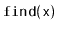method works by following the search path for the smallest value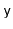such that:

```    Node<T> findPredNode(T x) {
Node<T> u = sentinel;
int r = h;
while (r >= 0) {
while (u.next[r] != null && compare(u.next[r].x,x) < 0)
u = u.next[r];   // go right in list r
r--;               // go down into list r-1
}
return u;
}
T find(T x) {
Node<T> u = findPredNode(x);
return u.next == null ? null : u.next.x;
}
```

Following the search path foris easy: when situated at some node,, in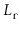, we look right to. If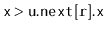, then we take a step to the right in; otherwise, we move down into. Each step (right or down) in this search takes only constant time; thus, by Lemma 4.1, the expected running time ofis.

Before we can add an element to a SkipListSSet, we need a method to simulate tossing coins to determine the height,, of a new node. We do so by picking a random integer,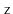, and counting the number of trailings in the binary representation of:4.1

```    int pickHeight() {
int z = rand.nextInt();
int k = 0;
int m = 1;
while ((z & m) != 0) {
k++;
m <<= 1;
}
return k;
}
```

To implement the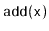method in a SkiplistSSet we search forand then spliceinto a few lists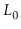,...,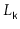, whereis selected using the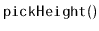method. The easiest way to do this is to use an array,, that keeps track of the nodes at which the search path goes down from some listinto. More precisely,is the node inwhere the search path proceeded down into. The nodes that we modify to insert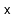are precisely the nodes. The following code implements this algorithm for:

```    boolean add(T x) {
Node<T> u = sentinel;
int r = h;
int comp = 0;
while (r >= 0) {
while (u.next[r] != null
&& (comp = compare(u.next[r].x,x)) < 0)
u = u.next[r];
if (u.next[r] != null && comp == 0) return false;
stack[r--] = u;          // going down, store u
}
Node<T> w = new Node<T>(x, pickHeight());
while (h < w.height())
stack[++h] = sentinel;   // height increased
for (int i = 0; i < w.next.length; i++) {
w.next[i] = stack[i].next[i];
stack[i].next[i] = w;
}
n++;
return true;
}
```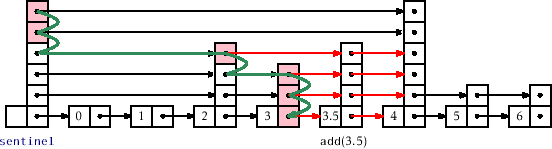Removing an element,, is done in a similar way, except that there is no need forto keep track of the search path. The removal can be done as we are following the search path. We search forand each time the search moves downward from a node, we check if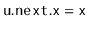and if so, we spliceout of the list:

```    boolean remove(T x) {
boolean removed = false;
Node<T> u = sentinel;
int r = h;
int comp = 0;
while (r >= 0) {
while (u.next[r] != null
&& (comp = compare(u.next[r].x, x)) < 0) {
u = u.next[r];
}
if (u.next[r] != null && comp == 0) {
removed = true;
u.next[r] = u.next[r].next[r];
if (u == sentinel && u.next[r] == null)
h--;  // height has gone down
}
r--;
}
if (removed) n--;
return removed;
}
```## 4.2.1 Summary

The following theorem summarizes the performance of skiplists when used to implement sorted sets:

Theorem 4..1   SkiplistSSet implements the SSet interface. A SkiplistSSet supports the operations,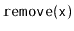, andinexpected time per operation.

#### Footnotes

...:4.1
This method does not exactly replicate the coin-tossing experiment since the value ofwill always be less than the number of bits in an. However, this will have negligible impact unless the number of elements in the structure is much greater than.
opendatastructures.org